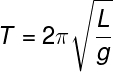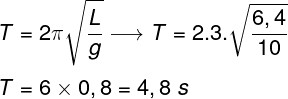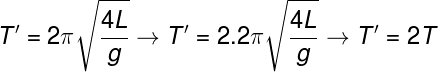Sound and Oscillation

# simple pendulum

The simple pendulum is a mechanical system that consists of a point mass, that is, a body with insignificant dimensions, attached to a string of negligible and inextensible mass capable of oscillating around a fixed position . Thanks to its simplicity, this pendulum is widely used during the study of simple harmonic motion .

## How does the simple pendulum work?

The simple pendulum is an approximation in which there are no dissipative forces , that is, frictional or dragging forces , acting on any components of the system. In these pendulums, the oscillatory movement arises as a result of the action of the forces of weight and traction , exerted by a string. Watch:

As the weight and traction forces do not cancel each other out in this context, as this only happens in the equilibrium position, a resultant force of a centripetal nature arises , causing the pendulum to oscillate around an equilibrium point.

From the hourly equations of simple harmonic motion and Newton ‘s laws , it is possible to determine a set of equations unique to simple pendulums , for this we say that the resultant between the weight force and the traction force is a centripetal force. Also, the restoring force of the pendulum motion is the horizontal component of the weight:

x – horizontal component of the weight force (N)

y – vertical component of the weight force (N)

## Simple pendulum formulas

The formula shown below is used to calculate the period in the simple pendulum, it relates the time of a complete swing to the size of the wire and the local acceleration of gravity , check:T – period (s)

L – wire length (m)

g – gravity (m/s²)

The previous formula shows us that the time of oscillation in the simple pendulum does not depend on the mass of the object that is oscillating. In order to derive this formula, it is necessary to assume that the oscillation occurs only at small angles , so that the sine of angle θ is very close to the value of θ itself, in degrees.

## Energy transformations in the simple pendulum

During the swing of the simple pendulum, the mechanical energy remains constant , however, with each complete swing, the exchange between kinetic and gravitational potential energies occurs . The energy transfer between the different positions of the pendulum as a function of time of an oscillation appears as a sine wave , as shown in the following figure:

In the figure above, we show three special pendulum positions. At positions 1 and 3, there is maximum gravitational potential energy and minimum kinetic energy , at position 2, there is maximum kinetic energy and minimum gravitational potential energy .

## Exercises solved

Question 1)  Determine the period of oscillation of a simple pendulum, knowing that the pendulum’s string is 6.4 m long. Assume the local gravity acceleration equal to 10 m/s² and use π = 3.

a) 0.64 s

b) 4.80 s

c) 4.16 s

d) 2.40 s

e) 0.48 s

alternative b.

Resolution

To solve the question, we will make use of the formula that calculates the period of oscillation of the simple pendulum, observe:The calculation made shows that the period of this oscillatory movement will be 3.84 s, so the correct alternative is letter b.

Question 2) A pendulum of length L is set to swing in a region where gravity is g. It is known that the period of each swing of this pendulum is T. If we were to quadruple the length of this pendulum, the new period, written in terms of the original period, would be:

a) T’ = 4T

b) T’ = T/2

c) T’ = 2T

d) T’ = T/4

e) T’ = T/16

alternative c.

Resolution

To solve the exercise, we just substitute the new length, four times greater than the previous length, L. Note:In the calculation shown above, the 4 that was inside the root becomes equal to 2, when written outside of it, so the correct alternative is the letter c.

Question 3) Regarding the movement performed by a point mass in a simple pendulum, mark the correct one:

a) In a simple pendulum, the kinetic energy remains constant throughout the swing.

b) In a simple pendulum, the mechanical energy remains constant throughout the swing.

c) In a simple pendulum, the speed remains constant throughout the swing.

d) In a simple pendulum, the period is directly proportional to the mass that is oscillating.

e) In a simple pendulum, the longer the length of the string, the shorter the period of oscillation.

Resolution

Alternative b , because the simple pendulum is an oscillatory movement in which the kinetic energy is interchangeable with the gravitational potential energy without energy losses, because in this movement, there are no dissipative forces, in this way, only the mechanical energy is kept constant.